# Manipulating DataFrames with Pandas – Python

• Last Updated : 31 May, 2021

Before manipulating the dataframe with pandas we have to understand what is data manipulation. The data in the real world is very unpleasant & unordered so by performing certain operations we can make data understandable based on one’s requirements, this process of converting unordered data into meaningful information can be done by data manipulation.

Here, we will learn how to manipulate dataframes with pandas. Pandas is an open-source library that is used from data manipulation to data analysis & is very powerful, flexible & easy to use tool which can be imported using import pandas as pd. Pandas deal essentially with data in 1-D and 2-D arrays; Although, pandas handles these two differently. In pandas, 1-D arrays are stated as a series & a dataframe is simply a 2-D array. The dataset used here is country_code.csv.

Below are various operations used to manipulate the dataframe:

• First, import the library which is used in data manipulation i.e. pandas then assign and read the dataframe:

## Python3

 `# import module``import` `pandas as pd` `# assign dataset``df ``=` `pd.read_csv(``"country_code.csv"``)` `# display``print``(``"Type-"``, ``type``(df))``df`

Output: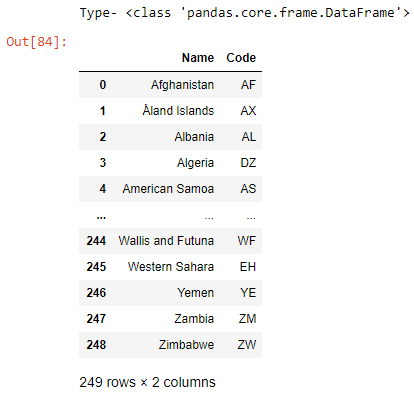• We can read the dataframe by using head() function also which is having an argument (n) i.e. number of rows to be displayed.

## Python3

 `df.head(``10``)`

Output: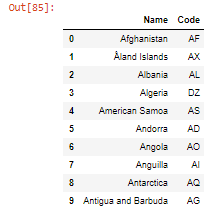• Counting the rows and columns in DataFrame using shape(). It returns the no. of rows and columns enclosed in a tuple.

## Python3

 `df.shape`

Output: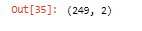• Summary of Statistics of DataFrame using describe() method.

## Python3

 `df.describe()`

Output: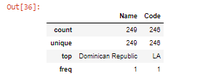• Dropping the missing values in DataFrame, it can be done using the dropna() method, it removes all the NaN values in the dataframe.

## Python3

 `df.dropna()`

Output: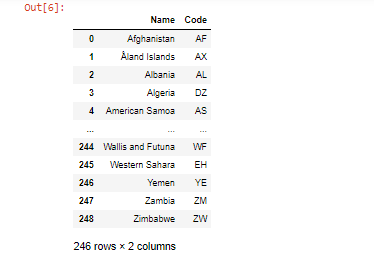Another example is:

## Python3

 `df.dropna(axis``=``1``)`

This will drop all the columns with any missing values.

Output: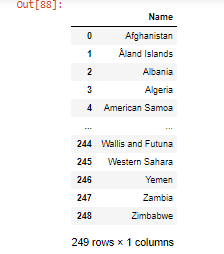• Merging DataFrames using merge(), arguments passed are the dataframes to be merged along with the column name.

## Python3

 `df1 ``=` `pd.read_csv(``"country_code.csv"``)``merged_col ``=` `pd.merge(df, df1, on``=``'Name'``)``merged_col`

Output: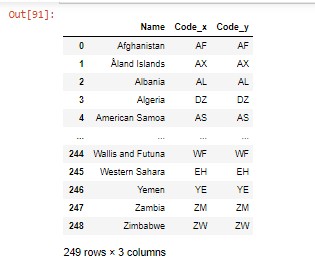• An additional argument ‘on’ is the name of the common column, here ‘Name’ is the common column given to the merge() function. df is the first dataframe & df1 is the second dataframe that is to be merged.
• Renaming the columns of dataframe using rename(), arguments passed are the columns to be renamed & inplace.

## Python3

 `country_code ``=` `df.rename(columns``=``{``'Name'``: ``'CountryName'``,``                                  ``'Code'``: ``'CountryCode'``},``                         ``inplace``=``False``)``country_code`

Output: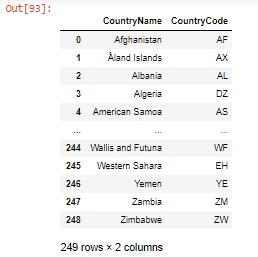The code ‘inplace = False’ means the result would be stored in a new DataFrame instead of the original one.

• Creating a dataframe manually:

## Python3

 `student ``=` `pd.DataFrame({``'Name'``: [``'Rohan'``, ``'Rahul'``, ``'Gaurav'``,``                                 ``'Ananya'``, ``'Vinay'``, ``'Rohan'``,``                                 ``'Vivek'``, ``'Vinay'``],``                        ` `                        ``'Score'``: [``76``, ``69``, ``70``, ``88``, ``79``, ``64``, ``62``, ``57``]})` `# Reading Dataframe``student`

Output: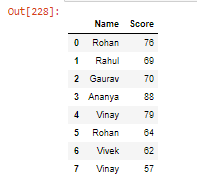• Sorting the DataFrame using sort_values() method.

## Python3

 `student.sort_values(by``=``[``'Score'``], ascending``=``True``)`

Output: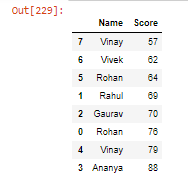• Sorting the DataFrame using multiple columns:

## Python3

 `student.sort_values(by``=``[``'Name'``, ``'Score'``],``                    ``ascending``=``[``True``, ``False``])`

Output: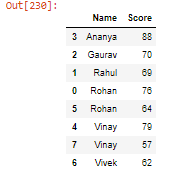• Creating another column in DataFrame, Here we will create column name percentage which will calculate the percentage of student score by using aggregate function sum().

## Python3

 `student[``'Percentage'``] ``=` `(student[``'Score'``] ``/` `student[``'Score'``].``sum``()) ``*` `100``student`

Output: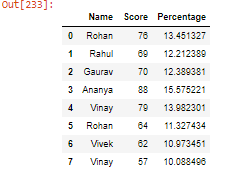• Selecting DataFrame rows using logical operators:

## Python3

 `# Selecting rows where score is``# greater than 70``print``(student[student.Score>``70``])` `# Selecting rows where score is greater than 60``# OR less than 70``print``(student[(student.Score>``60``) | (student.Score<``70``)])`

Output: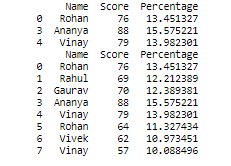• Indexing & Slicing :

Here .loc is label base & .iloc is integer position based methods used for slicing & indexing of data.

## Python3

 `# Printing five rows with name column only``# i.e. printing first 5 student names.``print``(student.loc[``0``:``4``, ``'Name'``])` `# Printing all the rows with score column``# only i.e. printing score of all the``# students``print``(student.loc[:, ``'Score'``])` `# Printing only first rows having name,``# score columns i.e. print first student``# name & their score.``print``(student.iloc[``0``, ``0``:``2``])` `# Printing first 3 rows having name,score &``# percentage columns i.e. printing first three``# student name,score & percentage.``print``(student.iloc[``0``:``3``, ``0``:``3``])` `# Printing all rows having name & score``# columns i.e. printing all student``# name & their score.``print``(student.iloc[:, ``0``:``2``])`

Output:

.loc: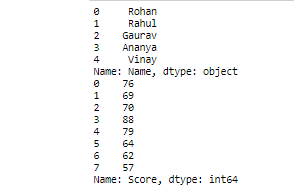.iloc: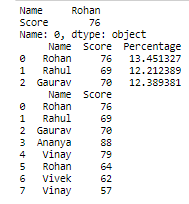• Apply Functions, this function is used to apply a function along an axis of dataframe whether it can be row (axis=0) or column (axis=1).

## Python3

 `# explicit function``def` `double(a):``    ``return` `2``*``a` `student[``'Score'``] ``=` `student[``'Score'``].``apply``(double)` `# Reading Dataframe``student`

Output: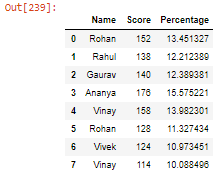My Personal Notes arrow_drop_up